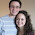## Thursday, April 25, 2013

### A case I hadn't given much thought to until recently

A recent run-in with an old AP Calculus question has got me thinking about relative extrema lately, specifically in the case of a removable discontinuity, as in this graph:Question:  If the graph above represents a function f, does f attain a relative minimum at x=b?

1.Yes, I think. We only require that f(b)<=f(x) on some open interval containing b, right?

And this doesn't throw off our first derivative test, either. We check places where the first derivative is zero or undefined (like it is here). If the derivative is undefined, we have to do a bit more careful analysis and check the actual value of the function.

I think this is similar to if you were finding extrema on y=abs(x) or y=x^(2/3). Or maybe piecewise-defined functions like your picture or like y=x (x>=0), y=-x+1 (x<0).

1.I agree-we have a relative min at x=b, as it does the follow the definition.

But, I don't think we can use the first derivative test here since f needs to be continuous at x=b in order to apply the test. So, in that sense, I wouldn't say it's similar to finding relative extrema that occur at corners or cusps. Or, what do you think?

2.Yeah, you're right, first derivative test is conditioned on continuity. Hmm.. I guess we're in the wild on this one, where anything goes! Maybe we can come up with some other pathological examples.

I guess the first derivative test isn't the be-all and end-all when looking for extrema. The most important thing is f(b)<=f(x) in some neighborhood of b. When in doubt, return to the definition!

3.Exactly. Definitions, definitions!

2.I found your blog as I was searching for the answer to a similar problem. I was using a removable discontinuity graph in the intro to my extrema lesson. I used y=x^2 with open circle at (0, 0) and the point located at (0, 1). I was trying to emphasize that (0, 0) is not an absolute min, but then the question of it being a relative max came up. According to the definition, I agree that it is a relative max. I wanted to find confirmation...I'm glad we agree.

Tell me what you think!